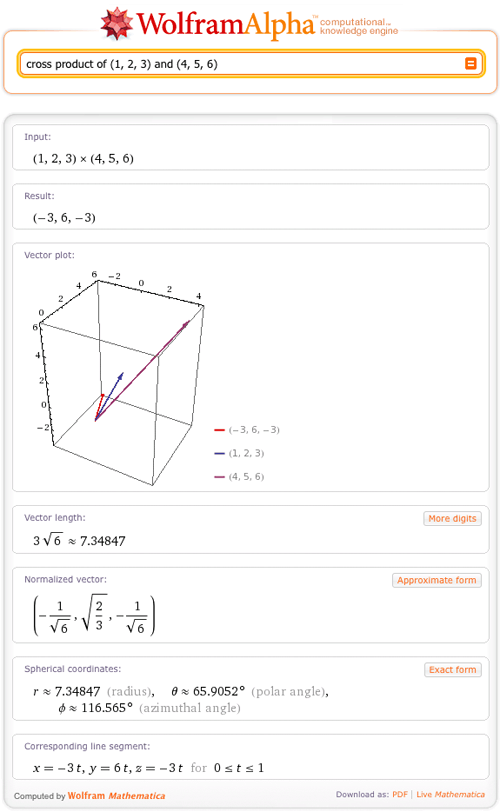AUTHOR PAGEErin Craig
Blog Posts from this author:
July 27, 2011–

In recent dinner conversation with my brother, I commented that I was “99.9 repeating” percent sure that my favorite author, Jorge Luis Borges, had lived into the 1980s (Wolfram|Alpha later showed me that he did, in fact, live through 1986!). I felt confident about my knowledge, but wanted to leave myself a little wiggle room, just in case. My brother grinned at me and said, “I know you know your geometric sequences. If you say you’re 99.9 repeating percent sure, then you’re 100 percent sure.”

I blushed, embarrassed. He was definitely right!

But just what did my brother mean? Well, he reminded me that:

99.999… = 99 + 9/10 + 9/100 + 9/1000 + 9/10000…

Glancing at the above, the series 9/10 + 9/100 + 9/1000 + 9/10000… stands out. Each term in this series is 1/10 times the term before it, making it a geometric series. A geometric series is a series wherein each term in the sequence is a constant number, r, multiplied by the term before it. Any geometric series whose r satisfies -1 < r < 1 is a convergent series, and we can say to what the series converges:

Sum[r^k, {k, 0, ∞}] = 1/(1-r)

June 1, 2011–

What do you get when you cross a mountain climber with a mosquito? Nothing—you can’t cross a scalar with a vector!

But what do you get when you cross two vectors? Wolfram|Alpha can tell you. For example:And in fact, Wolfram|Alpha can give lots of information on vectors. A vector is commonly defined as a quantity with both magnitude and direction and is often represented as an arrow. The direction of the arrow matches the direction of the vector, while the length represents the magnitude of the vector. Wolfram|Alpha can now plot vectors with this arrow representation in 2D and 3D and return many other properties of the vector.
More »

February 25, 2011–

Why did the mathematician name his dog Cauchy? Because he left a residue at every pole!

But could the mathematician find the poles and their residues for a given function? He certainly could, with the help of Wolfram|Alpha.

We are proud to announce that Wolfram|Alpha has added residues and poles to its ever-expanding library of mathematical data that it can compute! To showcase this behavior, let’s first recall just what a pole is.

In the study of complex analysis, a pole is a singularity of a function where the function behaves like 1/zn at z == 0 .

More technically, a point z_0 is a pole of a function if the Laurent series expansion of the function about the point z_0 has only finitely many terms with a negative degree of zz_0. As an example, let’s look at the Laurent expansion of 1/Sin[z] at z == 2 Pi: